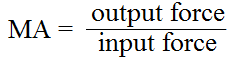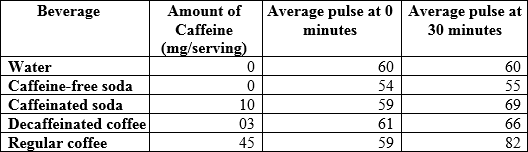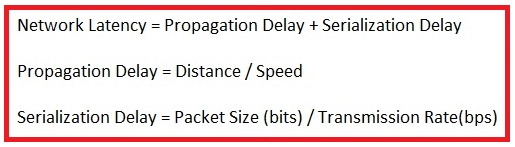# GED Science Practice Test 3

Directions: Choose the best answer to each question.

Congratulations - you have completed .

You scored %%SCORE%% out of %%TOTAL%%.

Your performance has been rated as %%RATING%%

 Question 1
Many individuals concerned with their carbon footprint are excited about the availability of hybrid electric vehicles. These cars are much more efficient in their use of fossil fuels than similarly sized internal combustion automobiles. However, hybrid electric vehicles do come with an environmental price tag. Their construction uses scarce metals, such as neodymium, lithium and lanthanum. Additionally, mining these elements involves pumping acids into deep boreholes to dissolve the surrounding rock and then removing the acid and mineral slurry. Such extraction processes are a type of surface mining, which have severe environmental impacts such as erosion, acid mine drainage, water pollution, and disruption of habitat.

### Based on the passage above, what are the environmental trade-offs of hybrid electric vehicles?

 A There is only enough lanthanum available in the world to meet the demand of the Toyota Prius. B While hybrid cars may reduce our dependence on fossil fuels, they may also increase our dependence on metals that are extracted from the ground in a destructive manner. C Water near surface mining operations is highly acidic, sometimes with a pH of 2.5 or lower. D Toyota obtains its lanthanum from China.
Question 1 Explanation:
Statement B explains that using hybrid electric vehicles will benefit the environment by reducing our dependence on fossil fuels, but it will come at a cost to the environment through the damaging methods that are required for the extraction of the limited resources these vehicles require. This information was provided in the passage.
 Question 2
How many calories does someone need to eliminate from their diet in order to lose weight? First they should calculate their basal metabolic rate (BMR) which identifies how many calories they burn each day when at complete rest. After calculating their BMR, they can then use an additional formula to factor in their level of activity. There are seperate BMR formulas for men and womnen, and these formulas require three variables: age, height, and current weight. Here is BMR formula for women:

BMR = 655 + (4.35 × W) × (4.7 x H) − (4.7 × A)

W = Weight in pounds
H = Height in inches
A = Age

### According to the formula, which of the following will result in a lower BMR?

 A Greater weight B Greater height C Greater age D None of the above
Question 2 Explanation:
From the equation, we can see that the age multiplied by 4.7 will be subtracted from the BMR. Thus, a higher number being multiplied by 4.7 will mean more is subtracted from the BMR, making it lower.
 Question 3

### Tonisha is conducting an experiment to determine the effects of watering clover with liquid Vitamin B. One week into her experiment the average clover plant from her control group is 17.3 millimeters tall.

(1 decimeter = 100 millimeters)
(1 meter = 10 decimeters)

### Convert this height measurement into meters:

 A 1.73 meters B 0.173 meters C 0.0173 meters D 0.00173 meters
Question 3 Explanation:
If you recall that there are 1000 millimeters in a meter, then you can simply divide 17.3 by 1000. You can also use the given information to do the conversion in two steps. First, convert to decimeters by dividing by 100 to get .173. Then, convert decimeters to meters by dividing by 10 to get a final answer of .0173.
 Question 4
Mechanical advantage is a measure of the force amplification that is achieved by using a tool, mechanical device, or machine system. The formula for mechanical advantage is:### A crowbar can be used to remove a nail from a board. If the crowbar has a mechanical advantage of 8, and the a force of 40 Newtons is applied to it, what will be the output force?

 A 5 Newtons B 320 Newtons C 0.2 Newtons D 32 Newtons
Question 4 Explanation:
The question provides the mechanical advantage and the input force. The first step is to plug these numbers into the equation:To solve this, multiply each side by 40:
8 × 40 = x
x = 320
 Question 5
Many individuals concerned with their carbon footprint are excited about the availability of hybrid electric vehicles. These cars are much more efficient in their use of fossil fuels than similarly sized internal combustion automobiles. However, hybrid electric vehicles do come with an environmental price tag. Their construction uses scarce metals, such as neodymium, lithium and lanthanum. Additionally, mining these elements involves pumping acids into deep boreholes to dissolve the surrounding rock and then removing the acid and mineral slurry. Such extraction processes are a type of surface mining, which have severe environmental impacts such as erosion, acid mine drainage, water pollution, and disruption of habitat.

### Which sentence from the passage above best supports the idea that driving a hybrid electric vehicle burns gasoline?

 A “Many individuals concerned with their carbon footprint are excited about the availability of hybrid electric vehicles.” B “These cars are much more efficient in their use of fossil fuels than similarly sized internal combustion automobiles.” C “However, hybrid electric vehicles do come with an environmental price tag” D “Such extraction processes are a type of surface mining, which have severe environmental impacts such as erosion, acid mine drainage, water pollution, and disruption of habitat.”
Question 5 Explanation:
The second sentence states that hybrid cars are "much more efficient in their use of fossil fuels..." This indicates that they do use at least some amount of fossil fuels (such as gasoline) in order to operate.
 Question 6

### In a lab experiment designed to test the rate at which plants grow under artificial light, the kind of light used is which type of variable?

 A Dependent B Independent C Random D Responding
Question 6 Explanation:
In an experiment, an independent variable is a factor controlled by the scientists. A dependent variable represents the output or effect. Since the light is controlled by the experimenters, it is an independent variable.
 Question 7
An experiment was performed to determine the effect of caffeine on the pulse rate of 20 healthy 20-year-old women. Each woman, in a state of fasting, was given a 250 mL beverage at the same time of day. The women had their pulse rates measured before they consumed the beverage, and then 30 minutes after consuming the beverage. This process was repeated over 5 days, with 5 different beverages. Below are the results:### After analyzing the data, what is the most appropriate conclusion about the effects of caffeine on the heart rate of healthy 20-year-old women?

 A Caffeine increases the pulse rates of women B Caffeine decreases the pulse rates of women C Caffeine is safe to consume D Regular coffee is healthier than decaffeinated coffee
Question 7 Explanation:
The results are conclusive that caffeine increases pulse rate, based on the fact that the caffeinated beverages all caused an increase in the pulse of the individuals tested.
 Question 8

### A student hypothesizes that eating a teaspoon of raw honey will prevent sneezing in people who are allergic to pollen. She runs an experiment to test her hypothesis. What is the dependent variable?

 A Eating B Raw Honey C Sneezing D Pollen
Question 8 Explanation:
An independent variable is a variable that is changed or controlled in a scientific experiment, in order to test the effects on the dependent variable. In this case the student is controlling the honey to test the effect on sneezing. It is called dependent because it "depends" on the independent variable.
 Question 9
A computer network is a set of computers connected together for the purpose of sharing resources. Network latency is an expression of how much time it takes for a packet of data to get from one designated point to another. For computer networks to work effectively, it is important to minimize network latency. The following equations can be used to calculate latency:### Which of the following changes would decrease network latency?

 A Increase distance B Decrease speed C Decrease packet size D Decrease transmission rate
Question 9 Explanation:
The first equation shows that network latency is the sum of propagation delay and serialization delay. So if you want to decrease network latency, you will need to decrease either of these delays.

The number above the line in a fraction is the numerator. Increasing the numerator will increase the value of the fraction. The number below the line in a fraction is the denominator. Increasing the denominator will decrease the value of the fraction.

To decrease network latency you need to either decrease a numerator or increase a denominator. Of the choices given, only a decrease in packet size will decrease a numerator.
 Question 10

### Purple flowers, yellow seeds and inflated seed pods are all dominant traits in pea plants. The recessive traits being white flowers, green seeds and constricted seed pods. If a plant that is homozygous dominant for flower color is crossed with a plant that is heterozygous for flower color, what percentage of the offspring will have yellow flowers?

 A 100% B 75% C 25% D 0%
Question 10 Explanation:
The question states that one plant is homozygous dominant for all three characters, meaning, that for each character, it has two dominant alleles. The genotype of this plant can be written as AABBCC. The other parent is heterozygous for flower color, meaning it has one dominant and one recessive allele, this genotype can be written as Aa. When an AA plant is crossed with an Aa plant there are two possible genotype outcomes, AA and Aa. Since the offspring will always receive at least one dominant allele none of the offspring would be expected to express the recessive flower color.
Once you are finished, click the button below. Any items you have not completed will be marked incorrect.
There are 10 questions to complete.
 ← List →